# Stable diffusion using 🤗 Hugging Face - DiffEdit paper implementation

Stable Diffusion
Research
Author

Aayush Agrawal

Published

November 17, 2022

An implementation of DIFFEDIT: DIFFUSION-BASED SEMANTIC IMAGE EDITING WITH MASK GUIDANCE using 🤗 hugging face diffusers library.

In this post, I am going to implement a recent paper that came from researchers in Meta AI and Sorbonne Universite named DIFFEDIT. This blog will make more sense to people who are either familiar with the stable diffusion process or are reading after four-part series I made on Stable Diffusion -
1. Part 1 - Stable diffusion using 🤗 Hugging Face - Introduction.
2. Part 2 - Stable diffusion using 🤗 Hugging Face - Looking under the hood.
3. Part 3 - Stable diffusion using 🤗 Hugging Face - Putting everything together
4. Part 4 - Stable diffusion using 🤗 Hugging Face - Variations of Stable Diffusion

Originally, this was the blog post I wanted to write about but realized there is no single place for understanding Stable diffusion with code. Which is the reason I ended up creating the four-part series as a reference or pre-read material to understand this paper.

## 1 What is DiffEdit?

In simple terms, you can think of DiffEdit approach as a more controlled version of the Image to Image pipeline. DiffEdit takes three inputs-
1. An input image
2. Caption - Describing the input image
3. Target Query - Describe the new image you want to generate

and produce a modified version of the original image based on the query text. This process is particularly good if you want to make a slight tweak to the actual image without completely modifying it.

As we can see from the image above only the fruits parts of the image were replaced with pears. Pretty amazing results!

The way the authors explain they achieve it is by introducing a mask generation module that determines which part of the image should be edited and then only perform text-based diffusion conditioning on the masked part.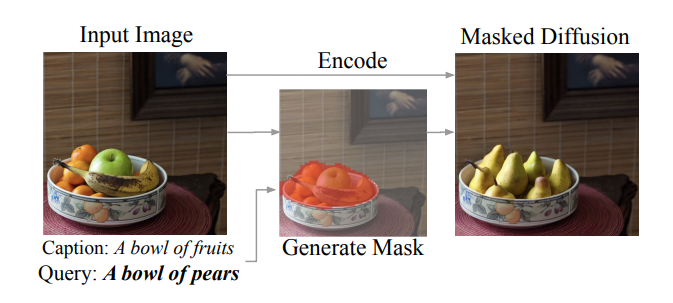Fig. 2: From the paper DiffEdit. An approach to change an input image by providing caption text and new text.

As we can see from the image above taken from the paper, the authors create a mask from the input image which accurately determines the part of the image where fruits are present and generate a mask (shown in Orange) and then perform masked diffusion to replace fruits with pears. Reading further the authors provide a good visual representation of the whole DiffEdit process.

As I was reading this paper, it seems generating the masking is the most important step and the rest is just textual conditioning using the diffusion process. The conditioning of an image using the mask is a similar idea implemented in Hugging face In-Paint Pipeline. As suggested by the authors, “there are three steps to the DiffEdit process -
Step 1: Add noise to the input image, and denoise it: once conditioned on the query text, and once conditioned on a reference text (or unconditionally). We derive a mask based on the difference in the denoising results.
Step2: we encode the input image with DDIM, to estimate the latents corresponding to the input image
Step3: we perform DDIM decoding conditioned on the text query, using the inferred mask to replace the background with pixel values coming from the encoding process at the corresponding timestep”1

In the next sections, we will start implementing these ideas into actual code.

Let’s start by importing the required libraries and helper functions. All of this was already used and explained in the previous part 2 and part 3 of the stable diffusion series.

Code
import torch, logging

## disable warnings
logging.disable(logging.WARNING)

## Imaging  library
from PIL import Image
from torchvision import transforms as tfms

## Basic libraries
import numpy as np
from tqdm.auto import tqdm
import matplotlib.pyplot as plt
%matplotlib inline
from IPython.display import display
import shutil
import os

## For video display
from IPython.display import HTML
from base64 import b64encode

## Import the CLIP artifacts
from transformers import CLIPTextModel, CLIPTokenizer
from diffusers import AutoencoderKL, UNet2DConditionModel, DDIMScheduler

## Helper functions

'''
A function to load all diffusion artifacts
'''
vae = AutoencoderKL.from_pretrained("CompVis/stable-diffusion-v1-4", subfolder="vae", torch_dtype=torch.float16).to("cuda")
unet = UNet2DConditionModel.from_pretrained("CompVis/stable-diffusion-v1-4", subfolder="unet", torch_dtype=torch.float16).to("cuda")
tokenizer = CLIPTokenizer.from_pretrained("openai/clip-vit-large-patch14", torch_dtype=torch.float16)
text_encoder = CLIPTextModel.from_pretrained("openai/clip-vit-large-patch14", torch_dtype=torch.float16).to("cuda")
scheduler = DDIMScheduler(beta_start=0.00085, beta_end=0.012, beta_schedule="scaled_linear", clip_sample=False, set_alpha_to_one=False)
return vae, unet, tokenizer, text_encoder, scheduler

'''
Function to load images from a defined path
'''
return Image.open(p).convert('RGB').resize((512,512))

def pil_to_latents(image):
'''
Function to convert image to latents
'''
init_image = tfms.ToTensor()(image).unsqueeze(0) * 2.0 - 1.0
init_image = init_image.to(device="cuda", dtype=torch.float16)
init_latent_dist = vae.encode(init_image).latent_dist.sample() * 0.18215
return init_latent_dist

def latents_to_pil(latents):
'''
Function to convert latents to images
'''
latents = (1 / 0.18215) * latents
image = vae.decode(latents).sample
image = (image / 2 + 0.5).clamp(0, 1)
image = image.detach().cpu().permute(0, 2, 3, 1).numpy()
images = (image * 255).round().astype("uint8")
pil_images = [Image.fromarray(image) for image in images]
return pil_images

def text_enc(prompts, maxlen=None):
'''
A function to take a texual promt and convert it into embeddings
'''
if maxlen is None: maxlen = tokenizer.model_max_length
inp = tokenizer(prompts, padding="max_length", max_length=maxlen, truncation=True, return_tensors="pt")
return text_encoder(inp.input_ids.to("cuda")).half()

vae, unet, tokenizer, text_encoder, scheduler = load_artifacts()

Let’s also download an image which we will use for the code implementation process.

p = FastDownload().download('https://images.pexels.com/photos/1996333/pexels-photo-1996333.jpeg?cs=srgb&dl=pexels-helena-lopes-1996333.jpg&fm=jpg&_gl=1*1pc0nw8*_ga*OTk4MTI0MzE4LjE2NjY1NDQwMjE.*_ga_8JE65Q40S6*MTY2Njc1MjIwMC4yLjEuMTY2Njc1MjIwMS4wLjAuMA..')
init_img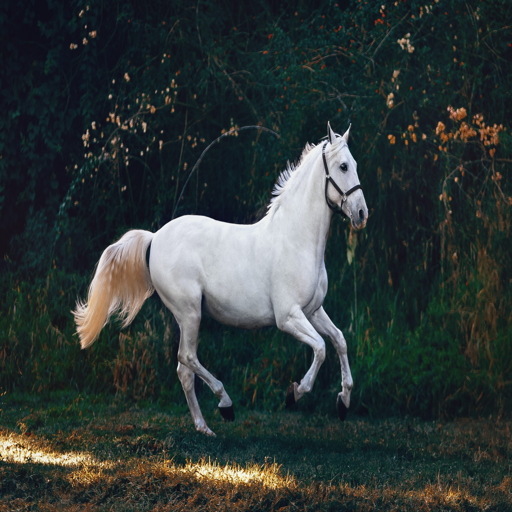## 2 DiffEdit: Purist implementation

Let’s start by implementing the paper as closely as the authors suggested, hence the Purist implementation.

### 2.1 Mask Creation: First Step of the DiffEdit process

There is a more detailed explanation of Step 1 from the paper, here are the key parts mentioned -
1. Denoise image using different text conditioning, one using reference text and the other using query text, and take differences from the result. The idea is there are more changes in the different parts and not in the background of the image.
2. Repeat this differencing process 10 times
3. Average out these differences and binarize for mask

Note

The third step in mask creation (averaging and binarization) is not explained clearly in the paper and it took me a lot of experiments to get this right.

First, we will try to implement the paper exactly as it’s mentioned. We will modify the prompt_2_img_i2i function for this task to return latents instead of rescaled and decoded de-noised images.

def prompt_2_img_i2i(prompts, init_img, neg_prompts=None, g=7.5, seed=100, strength =0.8, steps=50, dim=512):
"""
Diffusion process to convert prompt to image
"""
# Converting textual prompts to embedding
text = text_enc(prompts)

# Adding an unconditional prompt , helps in the generation process
if not neg_prompts: uncond =  text_enc([""], text.shape)
else: uncond =  text_enc(neg_prompt, text.shape)
emb = torch.cat([uncond, text])

# Setting the seed
if seed: torch.manual_seed(seed)

# Setting number of steps in scheduler
scheduler.set_timesteps(steps)

# Convert the seed image to latent
init_latents = pil_to_latents(init_img)

# Figuring initial time step based on strength
init_timestep = int(steps * strength)
timesteps = scheduler.timesteps[-init_timestep]
timesteps = torch.tensor([timesteps], device="cuda")

# Adding noise to the latents
noise = torch.randn(init_latents.shape, generator=None, device="cuda", dtype=init_latents.dtype)
latents = init_latents

# Computing the timestep to start the diffusion loop
t_start = max(steps - init_timestep, 0)
timesteps = scheduler.timesteps[t_start:].to("cuda")

# Iterating through defined steps
for i,ts in enumerate(tqdm(timesteps)):
# We need to scale the i/p latents to match the variance
inp = scheduler.scale_model_input(torch.cat([latents] * 2), ts)

# Predicting noise residual using U-Net
with torch.no_grad(): u,t = unet(inp, ts, encoder_hidden_states=emb).sample.chunk(2)

# Performing Guidance
pred = u + g*(t-u)

# Conditioning  the latents
#latents = scheduler.step(pred, ts, latents).pred_original_sample
latents = scheduler.step(pred, ts, latents).prev_sample

# Returning the latent representation to output an array of 4x64x64
return latents.detach().cpu()

Next, we will make a create_mask function, which will take an initial image, reference prompt, and query prompt with the number of times we need to repeat the steps. In the paper, the author suggests that n=10 and a strength of 0.5 works well in their experimentation. Hence, the default for the function is adjusted to that. create_mask function performs the following steps -
1. Create two denoised latents, one conditioned on reference text and the second on query text, and take a difference of these latents
2. Repeat this step n times
3. Take an average of these differences and standardize
4. Pick a threshold of 0.5 to binarize and create a mask

def create_mask(init_img, rp, qp, n=10, s=0.5):
## Initialize a dictionary to save n iterations
diff = {}

## Repeating the difference process n times
for idx in range(n):
## Creating denoised sample using reference / original text
orig_noise = prompt_2_img_i2i(prompts=rp, init_img=init_img, strength=s, seed = 100*idx)
## Creating denoised sample using query / target text
query_noise = prompt_2_img_i2i(prompts=qp, init_img=init_img, strength=s, seed = 100*idx)
## Taking the difference
diff[idx] = (np.array(orig_noise)-np.array(query_noise))

## Taking an average of 10 iterations
for idx in range(n):
## Note np.abs is a key step

## Averaging multiple channels

## Normalizing

## Binarizing and returning the mask object

mask = create_mask(init_img=init_img, rp=["a horse image"], qp=["a zebra image"], n=10)

Let’s visualize the generated mask over the image.

Code
plt.imshow(np.array(init_img), cmap='gray') # I would add interpolation='none'
plt.imshow(
cmap='cividis',
)
<matplotlib.image.AxesImage at 0x7ff6be5216d0>As we can see above, the mask produced covers the horse portion well which is what we want.

### 2.2 Masked Diffusion: Step 2 and 3 of DiffEdit paper.

Steps 2 and 3 need to be implemented in the same loop. Simply put author is saying to condition the latents based on reference text for the non-masked part and on query text for the masked part.
Combine these two parts using this simple formula to create combined latents -

$\hat{y}_{t} = My_{t} + (1-M)x_{t}$

def prompt_2_img_diffedit(rp, qp, init_img, mask, g=7.5, seed=100, strength =0.7, steps=70, dim=512):
"""
Diffusion process to convert prompt to image
"""
# Converting textual prompts to embedding
rtext = text_enc(rp)
qtext = text_enc(qp)

# Adding an unconditional prompt , helps in the generation process
uncond =  text_enc([""], rtext.shape)
emb = torch.cat([uncond, rtext, qtext])

# Setting the seed
if seed: torch.manual_seed(seed)

# Setting number of steps in scheduler
scheduler.set_timesteps(steps)

# Convert the seed image to latent
init_latents = pil_to_latents(init_img)

# Figuring initial time step based on strength
init_timestep = int(steps * strength)
timesteps = scheduler.timesteps[-init_timestep]
timesteps = torch.tensor([timesteps], device="cuda")

# Adding noise to the latents
noise = torch.randn(init_latents.shape, generator=None, device="cuda", dtype=init_latents.dtype)
latents = init_latents

# Computing the timestep to start the diffusion loop
t_start = max(steps - init_timestep, 0)
timesteps = scheduler.timesteps[t_start:].to("cuda")

# Converting mask to torch tensor

# Iterating through defined steps
for i,ts in enumerate(tqdm(timesteps)):
# We need to scale the i/p latents to match the variance
inp = scheduler.scale_model_input(torch.cat([latents] * 3), ts)

# Predicting noise residual using U-Net
with torch.no_grad(): u, rt, qt = unet(inp, ts, encoder_hidden_states=emb).sample.chunk(3)

# Performing Guidance
rpred = u + g*(rt-u)
qpred = u + g*(qt-u)

# Conditioning  the latents
rlatents = scheduler.step(rpred, ts, latents).prev_sample
qlatents = scheduler.step(qpred, ts, latents).prev_sample

# Returning the latent representation to output an array of 4x64x64
return latents_to_pil(latents)

Let’s visualize the generated image.

output = prompt_2_img_diffedit(
rp = ["a horse image"],
qp=["a zebra image"],
init_img=init_img,
g=7.5, seed=100, strength =0.5, steps=70, dim=512)

## Plotting side by side
fig, axs = plt.subplots(1, 2, figsize=(12, 6))
for c, img in enumerate([init_img, output]):
axs[c].imshow(img)
if c == 0 : axs[c].set_title(f"Initial image ")
else: axs[c].set_title(f"DiffEdit output")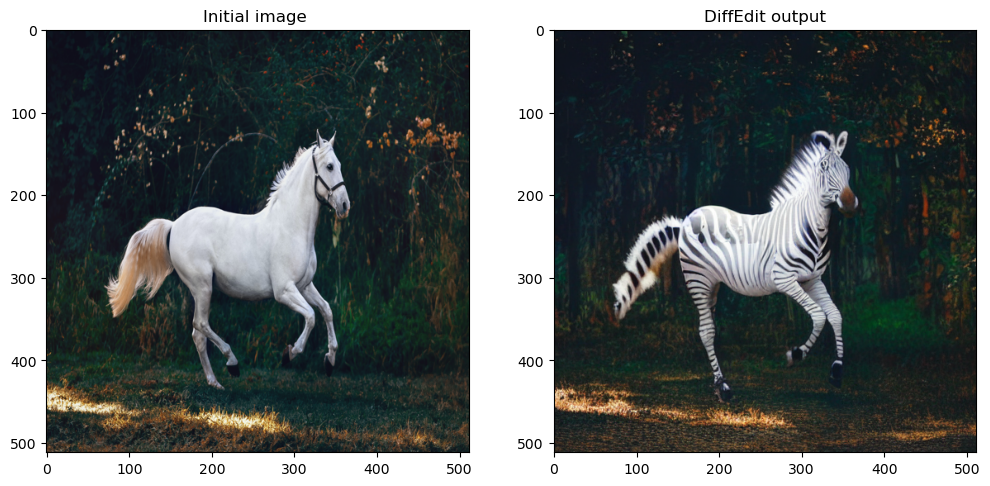Let’s create a simple function for the masking and diffusion process.

def diffEdit(init_img, rp , qp, g=7.5, seed=100, strength =0.7, steps=70, dim=512):

## Step 2 and 3: Diffusion process using mask
output = prompt_2_img_diffedit(
rp = rp,
qp=qp,
init_img=init_img,
g=g,
seed=seed,
strength =strength,
steps=steps,
dim=dim)
return mask , output

Let’s also create a visualization function for DiffEdit showing the original input image, masked image, and final output image.

def plot_diffEdit(init_img, output, mask):
## Plotting side by side
fig, axs = plt.subplots(1, 3, figsize=(12, 6))

## Visualizing initial image
axs.imshow(init_img)
axs.set_title(f"Initial image")

## Visualizing initial image
axs.imshow(output)
axs.set_title(f"DiffEdit output")

axs.imshow(np.array(init_img), cmap='gray')
axs.imshow(
cmap='cividis',
)
axs.set_title(f"DiffEdit mask")

Let’s test this function on a few images.

p = FastDownload().download('https://images.pexels.com/photos/1996333/pexels-photo-1996333.jpeg?cs=srgb&dl=pexels-helena-lopes-1996333.jpg&fm=jpg&_gl=1*1pc0nw8*_ga*OTk4MTI0MzE4LjE2NjY1NDQwMjE.*_ga_8JE65Q40S6*MTY2Njc1MjIwMC4yLjEuMTY2Njc1MjIwMS4wLjAuMA..')
mask, output = diffEdit(init_img, rp = ["a horse image"], qp=["a zebra image"])
plot_diffEdit(init_img, output, mask)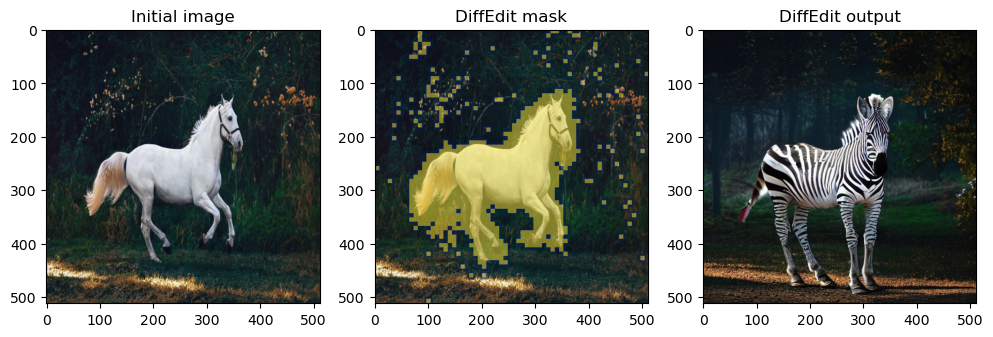Perfect, let’s try another one.

p = FastDownload().download('https://raw.githubusercontent.com/johnrobinsn/diffusion_experiments/main/images/bowloberries_scaled.jpg')
mask, output = diffEdit(init_img, rp = ['Bowl of Strawberries'], qp=['Bowl of Grapes'])
plot_diffEdit(init_img, output, mask)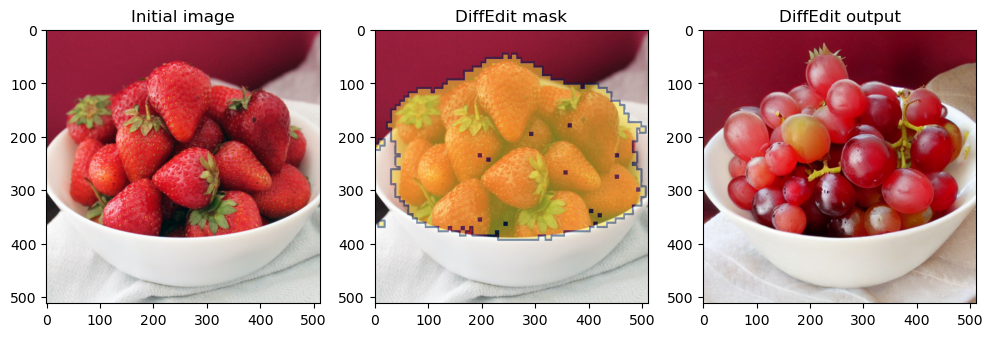## 3 FastDiffEdit: A faster DiffEdit implementation

Now we have seen the purist implementation, there are some improvements I suggest we can make to the original DiffEdit process in terms of speed and better results. Let’s call these improvements FastDiffEdit.

My biggest issue with the current way of doing masking is that it takes too much time(~50 sec on A4500 GPU). My take is we don’t need to run a full diffusion loop to denoise the image but just use the U-net prediction of the original sample in one shot and increase the repetition to 20 times. In this case, we can improve the computation from 10*25 = 250 steps to 20 steps (12x less loop). Let’s see if this works in practice.

def prompt_2_img_i2i_fast(prompts, init_img, g=7.5, seed=100, strength =0.5, steps=50, dim=512):
"""
Diffusion process to convert prompt to image
"""
# Converting textual prompts to embedding
text = text_enc(prompts)

# Adding an unconditional prompt , helps in the generation process
uncond =  text_enc([""], text.shape)
emb = torch.cat([uncond, text])

# Setting the seed
if seed: torch.manual_seed(seed)

# Setting number of steps in scheduler
scheduler.set_timesteps(steps)

# Convert the seed image to latent
init_latents = pil_to_latents(init_img)

# Figuring initial time step based on strength
init_timestep = int(steps * strength)
timesteps = scheduler.timesteps[-init_timestep]
timesteps = torch.tensor([timesteps], device="cuda")

# Adding noise to the latents
noise = torch.randn(init_latents.shape, generator=None, device="cuda", dtype=init_latents.dtype)
latents = init_latents

# We need to scale the i/p latents to match the variance
inp = scheduler.scale_model_input(torch.cat([latents] * 2), timesteps)
# Predicting noise residual using U-Net
with torch.no_grad(): u,t = unet(inp, timesteps, encoder_hidden_states=emb).sample.chunk(2)

# Performing Guidance
pred = u + g*(t-u)

# Zero shot prediction
latents = scheduler.step(pred, timesteps, latents).pred_original_sample

# Returning the latent representation to output an array of 4x64x64
return latents.detach().cpu()

Let’s create a new masking function that can take our prompt_2_img_i2i_fast function.

def create_mask_fast(init_img, rp, qp, n=20, s=0.5):
## Initialize a dictionary to save n iterations
diff = {}

## Repeating the difference process n times
for idx in range(n):
## Creating denoised sample using reference / original text
orig_noise = prompt_2_img_i2i_fast(prompts=rp, init_img=init_img, strength=s, seed = 100*idx)
## Creating denoised sample using query / target text
query_noise = prompt_2_img_i2i_fast(prompts=qp, init_img=init_img, strength=s, seed = 100*idx)
## Taking the difference
diff[idx] = (np.array(orig_noise)-np.array(query_noise))

## Taking an average of 10 iterations
for idx in range(n):
## Note np.abs is a key step

## Averaging multiple channels

## Normalizing

## Binarizing and returning the mask object
return (mask > 0).astype("uint8")

p = FastDownload().download('https://images.pexels.com/photos/1996333/pexels-photo-1996333.jpeg?cs=srgb&dl=pexels-helena-lopes-1996333.jpg&fm=jpg&_gl=1*1pc0nw8*_ga*OTk4MTI0MzE4LjE2NjY1NDQwMjE.*_ga_8JE65Q40S6*MTY2Njc1MjIwMC4yLjEuMTY2Njc1MjIwMS4wLjAuMA..')
plt.imshow(np.array(init_img), cmap='gray') # I would add interpolation='none'
plt.imshow(
cmap='cividis',
)
<matplotlib.image.AxesImage at 0x7ff6847a8b20>As we can see above the masking is improved and compute time has reduced from ~50 seconds to ~10 secs on my machine(5x improvement!).

Let’s improve our masking by adding a cv2 trick. This will just smooth out the masking a little bit more.

import cv2
return mask.astype('uint8')
mask = improve_mask(mask)
plt.imshow(np.array(init_img), cmap='gray') # I would add interpolation='none'
plt.imshow(
cmap='cividis',
)
<matplotlib.image.AxesImage at 0x7ff6a425caf0>As we can see above the masking has become a bit more smooth and covers more area.

### 3.2 Masked Diffusion: Replace with 🤗 inpaint pipeline

So, instead of using our function to perform the masked diffusion, there is a special pipeline in 🤗 diffusers library called inpaint pipeline. Which takes the query prompt, initial image, and generated mask to generate the output image. Let’s start by loading in the inpaint pipeline.

from diffusers import StableDiffusionInpaintPipeline
pipe = StableDiffusionInpaintPipeline.from_pretrained(
"runwayml/stable-diffusion-inpainting",
revision="fp16",
torch_dtype=torch.float16,
).to("cuda")

Let’s use the inpaint pipeline with our generated mask and image.

pipe(
prompt=["a zebra image"],
image=init_img,
generator=torch.Generator("cuda").manual_seed(100),
num_inference_steps = 20
).images
image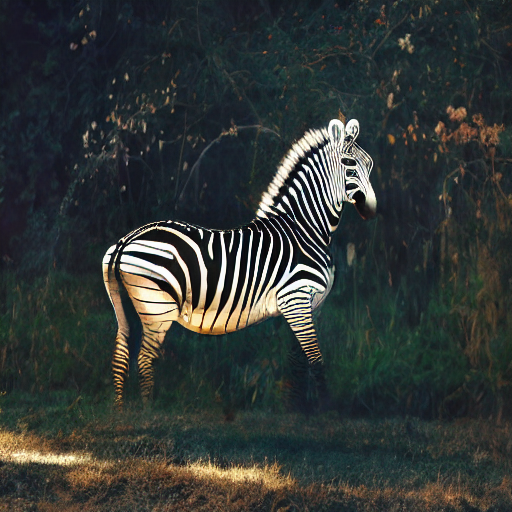As we can see above, inpaint pipeline creates a more realistic zebra image. Let’s create a simple function for the masking and diffusion process.

def fastDiffEdit(init_img, rp , qp, g=7.5, seed=100, strength =0.7, steps=20, dim=512):

## Improve masking using CV trick

## Step 2 and 3: Diffusion process using mask
output = pipe(
prompt=qp,
image=init_img,
generator=torch.Generator("cuda").manual_seed(100),
num_inference_steps = steps
).images
return mask , output

Let’s test this function on a few images.

p = FastDownload().download('https://images.pexels.com/photos/1996333/pexels-photo-1996333.jpeg?cs=srgb&dl=pexels-helena-lopes-1996333.jpg&fm=jpg&_gl=1*1pc0nw8*_ga*OTk4MTI0MzE4LjE2NjY1NDQwMjE.*_ga_8JE65Q40S6*MTY2Njc1MjIwMC4yLjEuMTY2Njc1MjIwMS4wLjAuMA..')
mask, output = fastDiffEdit(init_img, rp = ["a horse image"], qp=["a zebra image"])
plot_diffEdit(init_img, output, mask)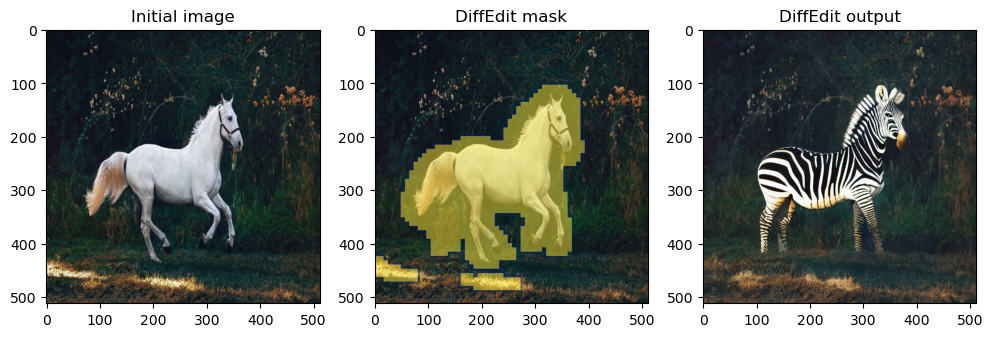Perfect, let’s try another one.

p = FastDownload().download('https://raw.githubusercontent.com/johnrobinsn/diffusion_experiments/main/images/bowloberries_scaled.jpg')
plot_diffEdit(init_img, output, mask)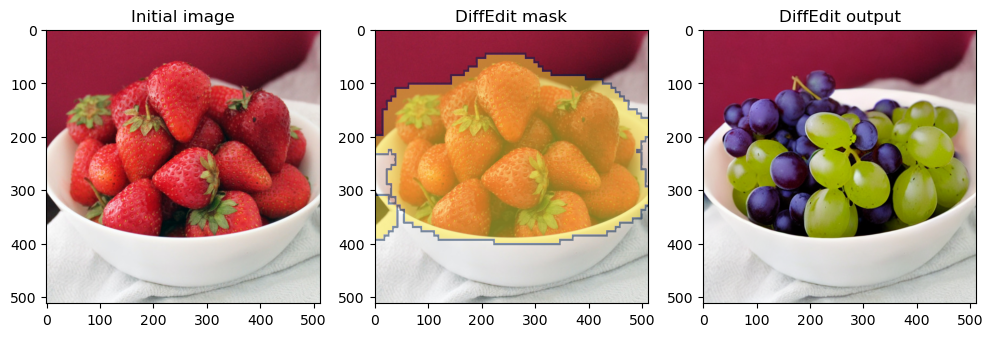In this post, we implemented the DiffEdit paper as the author mentioned and then we proposed improvements to the method to create FastDiffEdit which speeds up computation times up to 5 times.